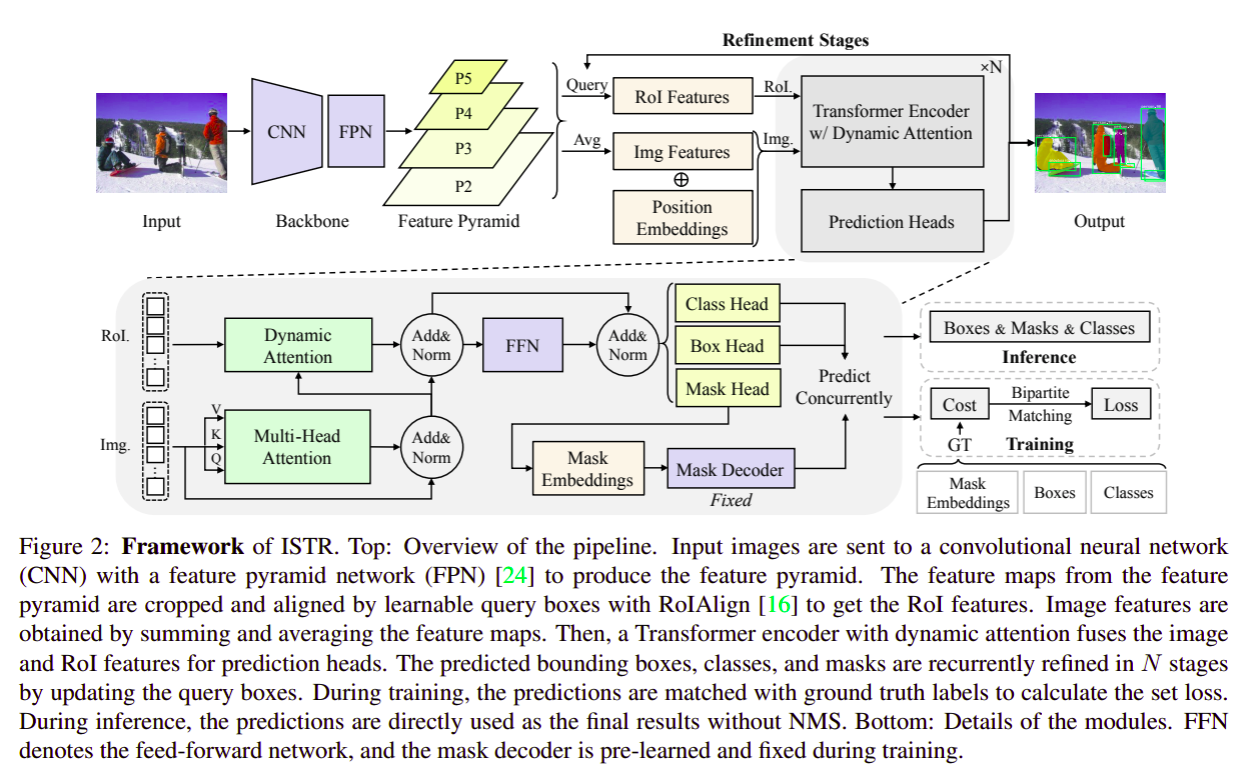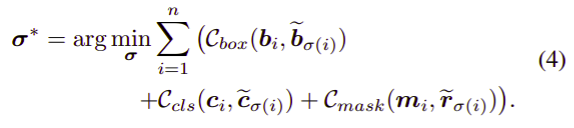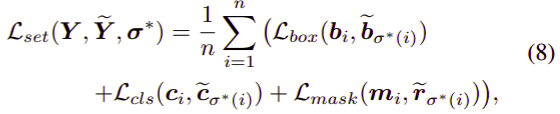# End-to-End Instance Segmentation

##### Posted on May 27, 20210 Comments

This note is for ISTR: End-to-End Instance Segmentation with Transformers.

End-to-end paradigms significantly improve the accuracy of various deep-learning-based computer vision models. - tasks like object detection have been upgraded by replacing non-end-to-end components - but such an upgrade is not applicable to instance segmentation, due to its significantly higher output dimensions compared to object detection.

The paper proposes an instance segmentation Transformer, termed ISTR, which is the first (??) end-to-end framework of its kind. - ISTR concurrently conducts detection and segmentation with a recurrent refinement strategy, which provides a new way to achieve instance segmentation compared to the existing top-down and bottom-up frameworks (??).

## Introduction

existing instance segmentation approaches: - either need a manually-designed post-processing step called non-maximum suppression (NMS) to remove duplicate predictions - or are early trials on small datasets and lack evaluation against modern baselines - popular approaches rely on a top-down or bottom-up framework that decomposes instance segmentation into several dependent tasks, preventing them from being end-to-end.

object detection also faces similar challenges: - recent studies enables end-to-end object detection by introducing a set prediction loss, with optimal use of Transformers. The set prediction loss enforces bipartite matching between labels and predictions to penalize redundant outputs, thus avoiding NMS during inference.

insufficient number of samples for learning the mask head: - the dimensions of masks are much higher that those of classes and boxes, e.g., a mask usually has a 28x28 or higher resolution on the COCO dataset, while a bounding box only needs two coordinates to represent. - the proposal bounding boxes obtained by the bipartite matching are usually on a small scale, which also raises the problem of sparse training samples. For example, Mask RCNN uses 512 proposal bounding boxes to extract the region of interest features for training the mask head, while the ground truths per image on the COCO dataset, is only 7.7 on average after bipartite matching.

Not all 28x28 entries are likely to appear as a mask. The distribution of the natural masks may lie in a low-dimensional manifold instead of being uniformly scattered. The paper propose to regress low-dimensional embeddings instead of raw masks.

### Instance Segmentation

it requires instance-level and pixel-level predictions.

Three categories of existing works: - top-down methods: first detect and then segment the objects - bottom-up methods: view instance segmentation as a label-then-cluster problem, learning to classify each pixel and then clustering them into groups for each object. - the latest work, SOLO, deals with instance segmentation without dependence on box detection. - the proposed ISTR provides a new perspective: directly predict a set of bounding boxes and mask embeddings, which avoids decomposing instance segmentation into dependent tasks. The idea of regressing mask embeddings is also in MEInst (To check).

### End-to-End Instance-Level Recognition

• the bipartite matching cost has become an essential component for achieving end-to-end object detection.
• some work explored the end-to-end mechanism with recurrent neural networks
• ISTR uses the similarity metric of mask embeddings as the bipartite matching cost for masks, and, for the first time, incorporates Transformers

### Transformers in Computer Vision

The breakthroughs of Transformers in natural language processing have sparked great interest in the computer vision community.

The critical component of Transformer is the multi-head attention, which can significantly enhance the capacity of models.

Transformers have been successfully used for

• image recognition
• object detection
• segmentation
• image superresolution
• video understanding
• image generation

A contemporary work achieves end-to-end video instance segmentation with a Transformer. Without continuous frames, the paper aims to segment instances for a single image.

• benefit of learning detection and segmentation jointly
• Mask R-CNN also demonstrated that bounding box detection could benefit from multi-task learning.

## Proposed Methodmaximize the mutual information between the original and reconstructed masks

$\max I(M, f(g(M)))$

where $M$ denotes a set of masks ${m_i}$, $g(\cdot)$ denotes the mask encoder for extracting embeddings and $f(\cdot)$ denotes the mask decoder for reconstructing masks.

A generalized objective function becomes

$\min \sum_{i=1}^n \Vert m_i - f(r_i) \Vert_2^2$

### Matching Cost and Prediction Loss

• the ground truth bounding boxes, classes, and masks: $Y={b_i, c_i, m_i\mid i=1,\ldots, n}$
• the predicting bounding boxes, classes, and mask embeddings: $\tilde Y={\tilde b_i, \tilde c_i, \tilde r_i\mid i=1,\ldots,k}$, where $k > n$

#### Bipartite Matching Cost#### Set Prediction Loss### Instance Segmentation Transformer

four main components:

• a CNN backbond with FPN: extract features for each instance
• a Transformer encoder with dynamic attention: learn the relation between objects
• a set of predicting heads: conduct detection and segmentation concurrently
• the $N$-step recurrent update for refining the set of predictions

Published in categories Note